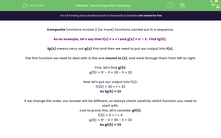# Use Composite Functions

In this worksheet, students will find outputs from composite functions involving up to three separate functions carried out in a set sequence.Key stage:  KS 4

Year:  GCSE

GCSE Subjects:   Maths

GCSE Boards:   Pearson Edexcel, OCR, Eduqas, AQA,

Curriculum topic:   Algebra

Curriculum subtopic:   Notation, Vocabulary and Manipulation Language of Functions

Difficulty level:#### Worksheet Overview

Composite functions involve 2 (or more) functions carried out in a sequence.

As an example, let's say that f(x) = x + 1 and g(x) = x² - 3.  Find fg(5).

fg(x) means carry out g(x) first and then we need to put our output into f(x).

The first function we need to deal with is the one closest to (x), and work through them from left to right.

First, let's find g(5):

g(5) = 5² - 3 = 25 - 3 = 22

Now let's put our output into f(x):

f(22) = 22 + 1 = 23

So fg(5) = 23

If we change the order, our answer will be different, so always check carefully which function you need to start with.

Just to prove this, let's consider gf(5).

f(5) = 5 + 1 = 6

g(6) = 6² - 3 = 36 - 3 = 33

So gf(5) = 33

In this activity, we will find outputs from composite functions involving up to three separate functions carried out in a sequence, correctly prioritising their order based on their proximity to (x).

### What is EdPlace?

We're your National Curriculum aligned online education content provider helping each child succeed in English, maths and science from year 1 to GCSE. With an EdPlace account you’ll be able to track and measure progress, helping each child achieve their best. We build confidence and attainment by personalising each child’s learning at a level that suits them.

Get started